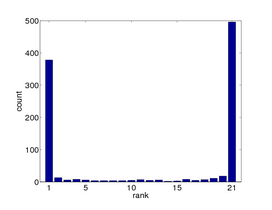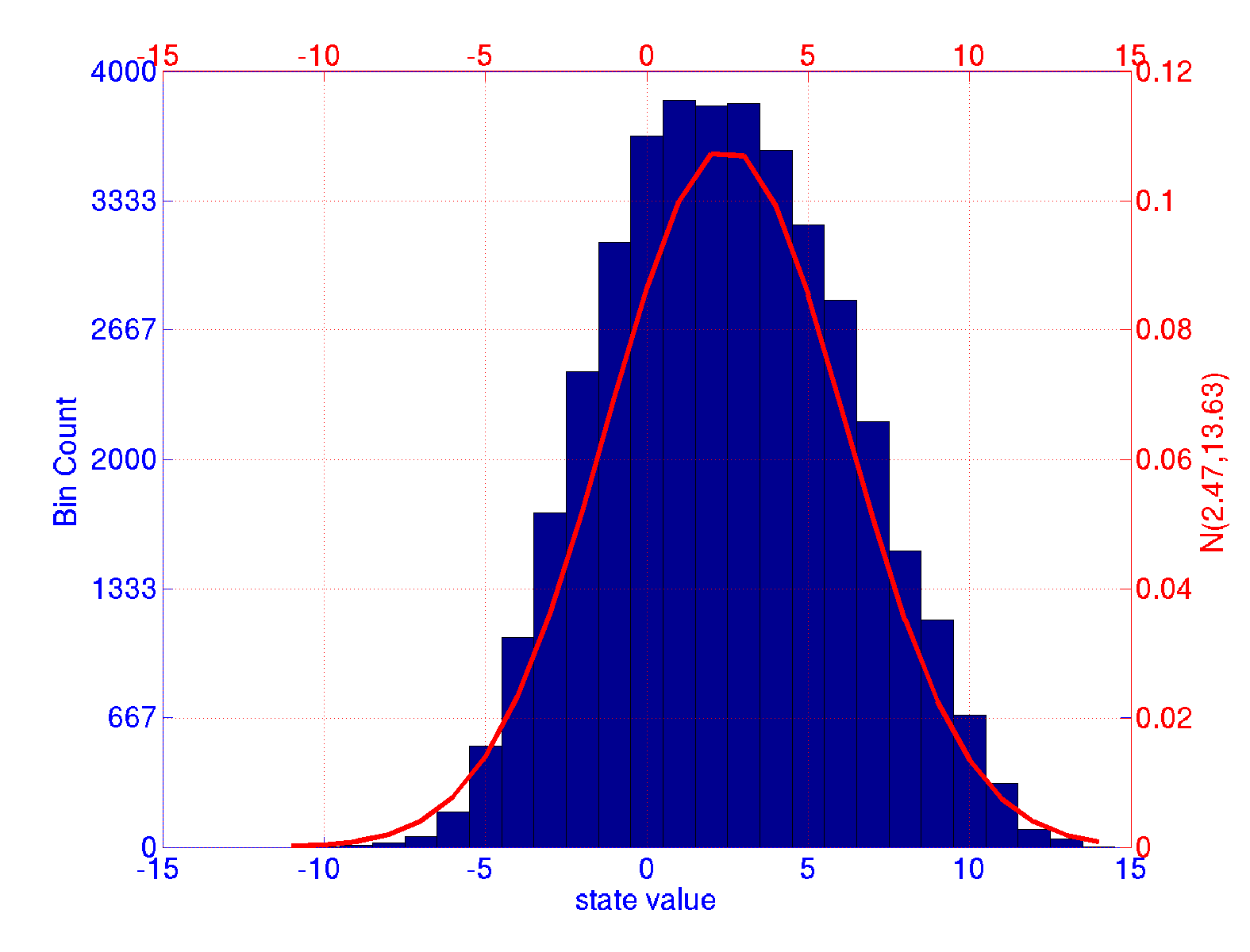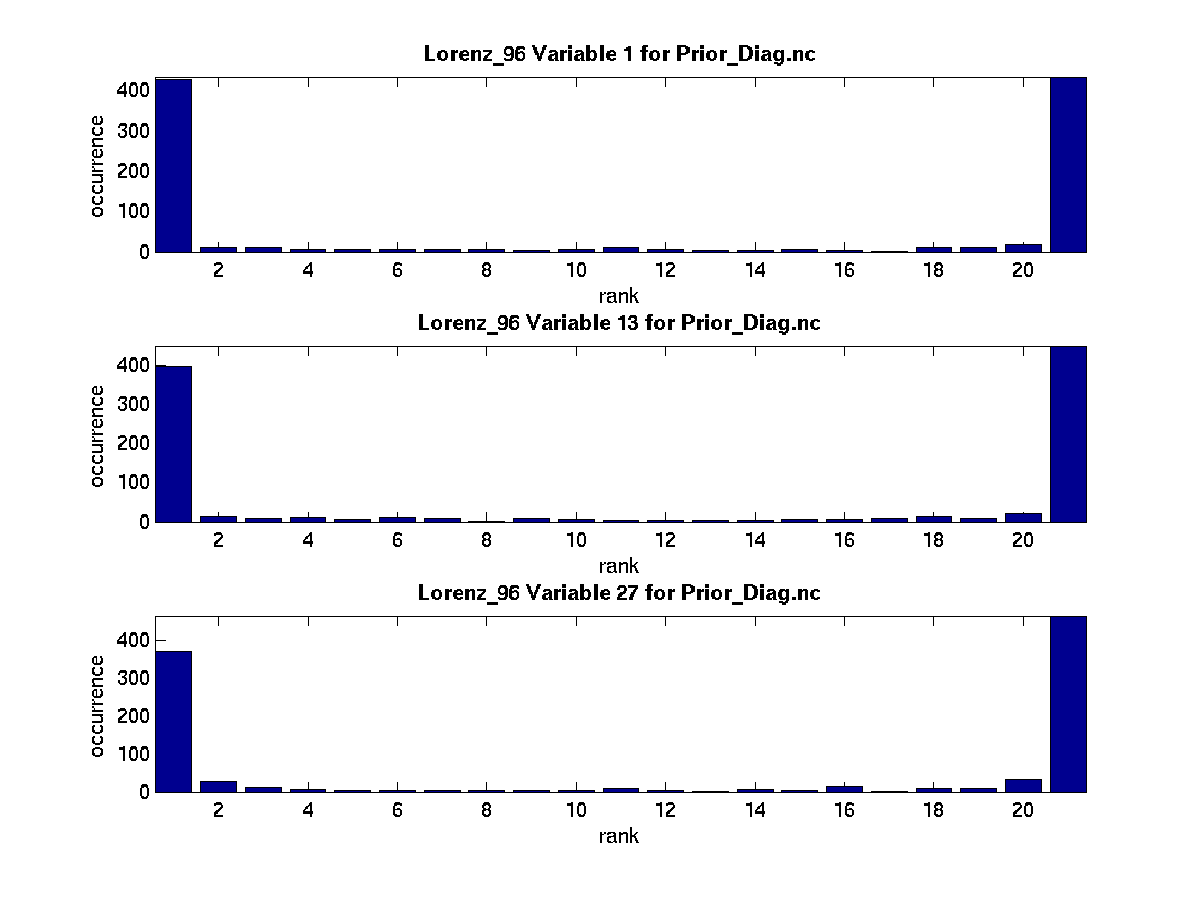# lorenz_96

Lorenz, E. N., and K. A. Emanuel, 1998:
Optimal sites for supplementary weather observations: Simulations with a small model.
J. Atmos. Sci.55, 399-414.
10.1175/1520-0469(1998)055<0399:OSFSWO>2.0.CO;2The Lorenz '96 model is one of our favorite models. In our implementation, it is a 40-variable model that can be used to test inflation algorithms, the effects of localization schemes, the integrity of the DART installation itself, the state-space diagnostic routines; it is extensively used in the tutorial, and can even be run as a standalone executable to test the MPI support on a machine. [link to more information] Jeff Anderson, jla@ucar.edu, and Tim Hoar, thoar@ucar.eduQuoting from the Lorenz 1998 paper:

... the authors introduce a model consisting of 40 ordinary differential equations, with the dependent variables representing values of some atmospheric quantity at 40 sites spaced equally about a latitude circle. The equations contain quadratic, linear, and constant terms representing advection, dissipation, and external forcing. Numerical integration indicates that small errors (differences between solutions) tend to double in about 2 days. Localized errors tend to spread eastward as they grow, encircling the globe after about 14 days.
...
We have chosen a model with J variables, denoted by X1, ..., XJ; in most of our experiments we have let J = 40. The governing equations are:
```dXj/dt = (Xj+1 - Xj-2)Xj-1 - Xj + F         (1)
```
for j = 1, ..., J. To make Eq. (1) meaningful for all values of j we define X-1 = XJ-1, X0 = XJ, and XJ+1 = X1, so that the variables form a cyclic chain, and may be looked at as values of some unspecified scalar meteorological quantity, perhaps vorticity or temperature, at J equally spaced sites extending around a latitude circle. Nothing will simulate the atmosphere's latitudinal or vertical extent.

# Document conventions

Anything underlined is a URL.
All filenames look like this -- (typewriter font, green).
Program names look like this -- (italicized font, green).
user input looks like this -- (bold, magenta).

commands to be typed at the command line are contained in an indented gray box.

And the contents of a file are enclosed in a box with a border:

&hypothetical_nml
obs_seq_in_file_name = "obs_seq.in",
obs_seq_out_file_name = "obs_seq.out",
init_time_days = 0,
init_time_seconds = 0,
output_interval = 1
&end

# Experiments/Exercises to run with the Lorenz '96 model.

Jeff Anderson has the most experience with the model.

• One Dimensional cyclic domain [0.0 1.0]
• Acts something like synoptic-scale weather around a mid-latitude circle.
• Attractor dimension ~ 13
• Start to explore model sizes closer to ensemble size
• Can examine possible degeneracy issues with sample covariance.
• Naive application of small ensembles diverge in many cases.

# Experiment 1 - defined in workshop_setup.csh

I recently had the pleasure of working with someone who had a funny twist on what I thought was a familiar expression: "You need to crawl before you can run.". He laughed and said in his culture they have an expression "You need to run and then crawl to catch your breath.". We're going to crawl FIRST.

This is a straighforward example of assimilating observations with an Ensemble Adjustment Kalman Filter for a pretty chaotic system. It is a spatially dense network (there are as many observations as state variables) which is observed 'frequently'. There is every reason to be optimistic, yet the result is dismal.

Running the canned example.
The following shell commands assume a 'pristine' directory - no modifications from the original distribution. All it takes to run this experiment is two simple commands:

cd DART/models/lorenz_96/work
./workshop_setup.csh

workshop_setup.csh is designed to compile all the DART programs for this model and run a predefined 'perfect model' experiment. We actually know the true trajectory (state) of the model and harvest observations (with some known noise characteristics) from the true state. We use an initial ensemble from around the true state and then assimilate these 'perfect' observations. Without the influence of observations, this model is sufficiently chaotic that each ensemble member (each copy of the model) would rapidly distance itself from the other members. (You can try that by assimilating an observation with an enormous error variance - fundamentally an uninformative observation.) Because all the ensemble members are being influenced by the observations, they tend to behave generally similar.

Knowing the Truth
It wouldn't be much of an illustration if we could not compare to the truth to see how we did.workshop_setup.csh is designed to compile all the DART programs for this model and run two programs: perfect_model_obs which generates the true state and corresponding observations, and filter which performs an assimilation using those observations. In this case, the experiment is to assimilate 40 randomly-located observations every timestep for 1000 timesteps. The observations have an error distribution N(0, 1); the True State is ~ N(2.47, 13.63) ... these are not particularly 'noisy' observations. The graphic to the left is a simple histogram of all of the values of all of the state variables for all 1000 timesteps. The curve depicts a normal pdf with the stated parameters evaluated at the centers of the bins of the histogram.

The DART program perfect_model_obs starts from an initial state (perfect_ics) and advances this state to the times declared in the observation sequence file obs_seq.in (don't worry about where this came from). When the model has reached the observation time, a simple forward operator is applied to the state vector to produce the 'perfect' observation, and a bit of random noise ~ N(0,1) is added to create the observations we actually assimilate - stored in a file named obs_seq.out. DART also records the true model trajectory in a netCDF file called True_State.nc. So - we know the true trajectory of the model, the 'perfect' observations, the 'synthetic' observations, AND the nature of the noise we added to those observations.

After we generate the truth and corresponding observations, it is time to assimilate.

The run-time control of the behavior of DART is through the Fortran namelist mechanism. All the namelists for DART are expected to be in a file named input.nml. Since the same system is used to assimilate very large models on supercomputers as well as our toy model on a laptop, there are many namelist parameters. I will only discuss the pertinent few.

• filter_nml: ens_size = 20 means to use 20 ensemble members. Since filter_nml: start_from_restart = .true., and filter_nml: restart_in_file_name = "filter_ics"; this file better have 20 sets of initial conditions in it - or DART will die and tell you why.
• filter_nml: inf_flavor = 0 use no inflation scheme.
• assim_tools_nml: filter_kind = 1 means an Ensemble Adjustment Kalman Filter (EAKF) will be used.
So - after running perfect_model_obs, workshop_setup.csh ran filter, which actually does the data assimilation. filter is the program that echoed a lot of stuff to the terminal window and ended with something like:
``` ...
...
Starting advance time loop
move_ahead  Start time of obs range day=  41 , sec=  55801
move_ahead  End time of obs range day=    41 , sec=  59400
Starting advance time loop
write_obs_seq  opening formatted file obs_seq.final
write_obs_seq  closed file obs_seq.final

--------------------------------------
Finished ... at YYYY MM DD HH MM SS =
2008 11 10 14 13  1
\$URL\$
\$Revision\$
\$Date\$
--------------------------------------
```
The (default) observation sequence file for the Lorenz96 model has 1000 timesteps in it. Since each timestep is 1 hour, it spans a time of 41 days 57600 seconds. For each timestep, all the observations within ± half of the timestep are assimilated. filter creates several output files:
• Prior_Diag.nc which contains the entire time evolution of every ensemble member - just before the data assimilation stage,
• Posterior_Diag.nc which contains the entire time evolution of every ensemble member - just after the data assimilation stage,
• obs_seq.final.nc which contains the entire observation sequence as well as each estimate of the observation from every ensemble member. This is crucial. The same operator that was applied to the true state to create the observation value is applied to each ensemble member (each 'copy' of the model state)

OK - so ... Did it Work?

DART comes with a set of Matlab® scripts and function that can be used to explore the results of an experiment. There are two broad avenues of exploration. For perfect model experiments, we know the True State (in True_State.nc) and we have the states of all the copies/ensemble members in Prior_Diag.nc and Posterior_Diag.nc. We could compare in state-space. In general, we don't know the true state, but we always have observations. We have the estimates of the observations from each of the ensemble members. We could compare in observation-space.

The Matlab® scripts to compare in state-space are in DART/matlab and the scripts to compare in observation-space are in DART/diagnostics/matlab. Since we have a perfect model experiment, lets get familiar with the state-space scripts in DART/matlab.

 plot_total_err.m is the Matlab script to plot the total error of the experiment. From the Matlab prompt, help plot_total_err will provide usage notes. You can compare the Posterior (after assimilation - which is pretty much cheating) model state or you can and should compare the Prior (in DART's case - a 1 step forecast) model state. In the perfect model scenario, we know the True State, so we just calculate the RMS error of the ensemble members at every state location and track that through time. It starts out with some initial value and by day 5 it gets much bigger and then levels off. Is this the best we can do?click on image for full-size versionclick on image for full-size version plot_ens_time_series.m is the Matlab script to plot the time evolution of the experiment. This script requires a user-defined state variable of interest - in this case, I just chose state variable index 1 (out of 40). The corresponding image chronicles the first 5 days of the experiment. Again, the Prior (also called the forecast or the guess) is compared to the known True State. The individual ensemble members are plotted in green, the ensemble mean is in red, and the true state is in blue. For a successful assimilation, it would be hoped the true state would be indistinguishable from the ensemble. Up through day 2, things are looking pretty good, but then the wheels fall off. The true state is no longer encompassed by the ensemble. By day 4.5, the ensemble is well separated from the true state. You could argue the ensemble spread is not large enough to encompass the truth; the end of that logic is that an ensemble with infinite variance would surely cover the truth - and would be totally useless. So, somehow we want an ensemble that has the smallest variance that is still indistinguishable from the truth.

Take a look around day 1. The True State is in the middle of the ensemble, and then near the edge. You could quantify this by determining the rank of the True State in the ensemble. If it is in the middle, there would be 10 ensemble members above it, 10 below. Right around day 1, there is only 1 ensemble member above the True State and 19 below. This introduces the next graphic, designed to summarize the rank of the True State for a particular ensemble size.click on image for full-size version plot_bins.m is the Matlab script to plot the rank histogram of the experiment. With an ensemble size of 20, there are 19 bins between the highest and lowest ensemble members. There are two more bins, one for anything above the highest and one for anything below the lowest. For each timestep in the experiment, simply sort the values of the ensemble and determine which bin would correspond to the value of the True State. Aggregate this count for the entire experiment and plot it as a histogram. If the True State is indistinguishable from the ensemble, the histogram should be pretty flat. Just because I could, and to prove it was happening to more than just one state variable, I plotted up 3 evenly-spaced state variables. It appears our True State is frequently outside the range spanned by the ensemble and makes our histogram decidedly 'U'-shaped. This means the assimilation is not good.

OK - so it did not work. What now?

Things to try:

• Increase the ensemble size. [link to experiment]
• Try a different filter algorithm. [link to experiment]
• Use some sort of inflation. [link to experiment]
• More observations. [link to experiment]